# Tag: Riemann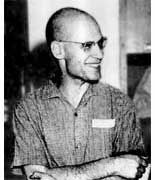The Oscar in
the category The Best Rejected Research Proposal in Mathematics
(ever)
goes to … Alexander Grothendieck
for his proposal Esquisse d’un Programme, Grothendieck\’s research program from 1983, written as
part of his application for a position at the CNRS, the French
equivalent of the NSF. An English translation is
available.

Here is one of the problems discussed :
Give TWO non-trivial elements of
$Gal(\overline{\mathbb{Q}}/\mathbb{Q})$
the _absolute_
Galois group of the algebraic closure of the rational numbers
$\overline{\mathbb{Q}}$, that is the group of all
$\mathbb{Q}$-automorphisms of $\overline{\mathbb{Q}}$. One element
most of us can give (complex-conjugation) but to find any other
element turns out to be an extremely difficult task.

To get a handle on
this problem, Grothendieck introduced his _’Dessins d’enfants’_
(Children’s drawings). Recall from last session the pictures of the
left and right handed Monsieur Mathieu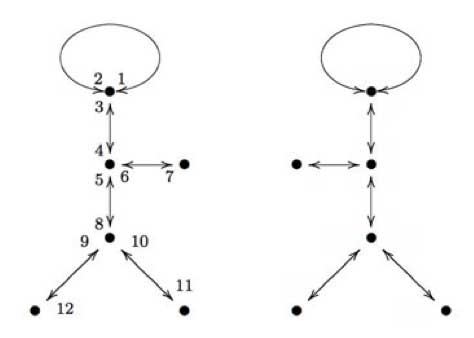The left hand side drawing was associated to a map
$\mathbb{P}^1_{\mathbb{C}} \rightarrow \mathbb{P}^1_{\mathbb{C}}$ which was
defined over the field $\mathbb{Q} \sqrt{-11}$ whereas the right side
drawing was associated to the map given when one applies to all
coefficients the unique non-trivial automorphism in the Galois group
$Gal(\mathbb{Q}\sqrt{-11}/\mathbb{Q})$ (which is
complex-conjugation). Hence, the Galois group
$Gal(\mathbb{Q}\sqrt{-11}/\mathbb{Q})$ acts _faithfully_ on the
drawings associated to maps $\mathbb{P}^1_{\mathbb{Q}\sqrt{-11}} \rightarrow \mathbb{P}^1_{\mathbb{Q}\sqrt{-11}}$ which are ramified only over
the points ${ 0,1,\infty }$.

Grothendieck’s idea was to
extend this to more general maps. Assume that a projective smooth curve
(a Riemann surface) X is defined over the algebraic numbers
$\overline{\mathbb{Q}}$ and assume that there is a map $X \rightarrow \mathbb{P}^1_{\mathbb{C}}$ ramified only over the points
${ 0,1,\infty }$, then we can repeat the procedure of last time and
draw a picture on X consisting of d edges (where d is the degree
of the map, that is the number of points lying over another point of
$\mathbb{P}^1_{\mathbb{C}}$) between white resp. black points (the
points of X lying over 1 (resp. over 0)).

Call such a drawing a
‘dessin d\’enfant’ and look at the collection of ALL dessins
d’enfants associated to ALL such maps where X runs over ALL curves
defined over $\overline{\mathbb{Q}}$. On this set, there is an action
of the absolute Galois group
$Gal(\overline{\mathbb{Q}}/\mathbb{Q})$ and if this action would be
faithful, then this would give us insight into this
group. However, at that time even the existence of a map $X \rightarrow \mathbb{P}^1$ ramified in the three points ${ 0,1,\infty }$
seemed troublesome to prove, as Grothendieck recalls in his proposal

In more erudite terms, could it be true that
every projective non-singular algebraic curve defined over a number
field occurs as a possible ‚ modular curve‚ parametrising
elliptic curves equipped with a suitable rigidification? Such a
supposition seemed so crazy that I was almost embarrassed to submit
it to the competent people in the domain. Deligne when I consulted
him found it crazy indeed, but didn’t have any counterexample up
his sleeve. Less than a year later, at the International Congress in
Helsinki, the Soviet mathematician Bielyi announced exactly that result,
with a proof of disconcerting simplicity which fit into two little
pages of a letter of Deligne ‚ never, without a doubt, was such a
deep and disconcerting result proved in so few lines!

In
the form in which Bielyi states it, his result essentially says that
every algebraic curve defined over a number field can be obtained as
a covering of the projective line ramified only over the points 0,
1 and infinity. This result seems to have remained more or less
unobserved. Yet, it appears to me to have considerable importance. To
me, its essential message is that there is a profound identity
between the combinatorics of finite maps on the one hand, and the
geometry of algebraic curves defined over number fields on the
other. This deep result, together with the algebraic- geometric
interpretation of maps, opens the door onto a new, unexplored world within reach of all, who pass by without seeing it.

Belyi’s proof is indeed relatively easy
(full details can be found in the paper Dessins d’enfants on the
Riemann sphere
by Leila
Schneps). Roughly it goes as follows : as both X and the map are
defined over $\overline{\mathbb{Q}}$ the map is only ramified over
(finitely many) $\overline{\mathbb{Q}}$-points. Let S be the finite
set of all Galois-conjugates of these points and consider the polynomial

$f_0(z_0) = \prod_{s \in S} (z_0 -s) \in \mathbb{Q}[z_0]$

Now, do a
resultant trick. Consider the
polynomial $f_1(z_1) = Res_{z_0}(\frac{d f_0}{d z_0},f_0(z_0)-z_1)$ then the roots of $f_1(z_1)$ are exactly the
finite critical values of $f_0$, $f_1$ is again defined over
$\mathbb{Q}$ and has lower degree (in $z_1$) than $f_0$ in $z_1$.
Continue this trick a finite number of times untill you have constructed
a polynomial $f_n(z_n) \in \mathbb{Q}[z_n]$ of degree zero.

Composing
the original map with the maps $f_j$ in succession yields that all
ramified points of this composition are
$\mathbb{Q}$-points! Now, we only have to limit the number of
these ramified $\mathbb{Q}$-points (let us call this set T) to three.

Take any three elements of T, then there always exist integers $m,n \in \mathbb{Z}$ such that the three points go under a linear
fractional transformation (a Moebius-function associated to a matrix in
$PGL_2(\mathbb{Q})$) to ${ 0,\frac{m}{m+n},1 }$. Under the
transformation $z \rightarrow \frac{(m+n)^{m+n}}{m^m n^n}z^m(1-z)^n$ the points 0 and 1 go to 0 and
$\frac{m}{m+n}$ goes to 1 whence the ramified points of the
composition are one less in number than T. Continuing in this way we
can get the set of ramified $\mathbb{Q}$-points of a composition at
most having three elements and then a final Moebius transformation gets
them to ${ 0,1,\infty }$, done!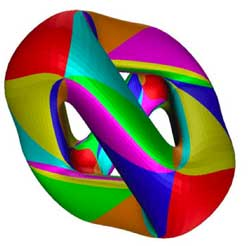As a tribute for this clever
argument, maps $X \rightarrow \mathbb{P}^1$ ramified only in 0,1 and
$\infty$ are now called Belyi morphisms. Here is an example of
a Belyi-morphism (and the corresponding dessin d’enfants) associated to
one of the most famous higher genus curves around : the Klein
quartic
(if you haven’t done
so yet, take your time to go through this marvelous pre-blog post by
John Baez).

One can define the Klein quartic as the plane projective
curve K with defining equation in
$\mathbb{P}^2_{\\mathbb{C}}$ given by $X^3Y+Y^3Z+Z^3X = 0$ K has
a large group of automorphism, namely the simple group of order
168 $G = PSL_2(\mathbb{F}_7) = SL_3(\mathbb{F}_2)$ It is a classical fact (see for example
the excellent paper by Noam Elkies The Klein quartic in number theory) that the quotient map $K \rightarrow K/G = \mathbb{P}^1_{\mathbb{C}}$ is ramified only in the points
0,1728 and $\infty$ and the number of points of K lying over them
are resp. 56, 84 and 24. Now, compose this map with the Moebius
transormation taking ${ 0,1728,\infty } \rightarrow { 0,1,\infty }$
then the resulting map is a Belyi-map for the Klein quartic. A
topological construction of the Klein quartic is fitting 24 heptagons
together so that three meet in each vertex, see below for the gluing
data-picture in the hyperbolic plane : the different heptagons are given
a number but they appear several times telling how they must fit
together)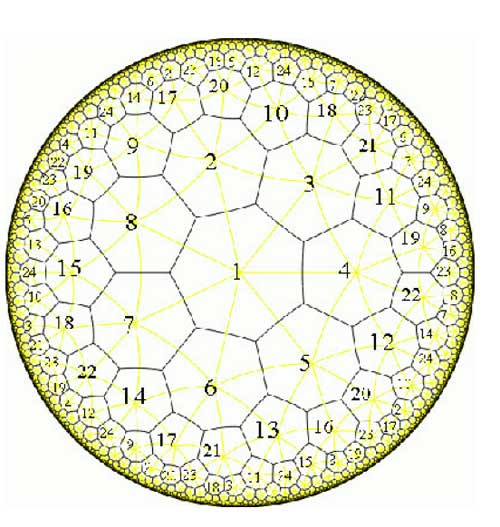The resulting figure has exactly $\frac{7 \times 24}{2} = 84$ edges and the 84 points of K lying over 1 (the white points in
the dessin) correspond to the midpoints of the edges. There are exactly
$\frac{7 \times 24}{3}=56$ vertices corresponding to the 56 points
lying over 0 (the black points in the dessin). Hence, the dessin
d\’enfant associated to the Klein quartic is the figure traced out by
the edges on K. Giving each of the 168 half-edges a
different number one assigns to the white points a permutation of order
two and to the three-valent black-points a permutation of order three,
whence to the Belyi map of the Klein quartic corresponds a
168-dimensional permutation representation of $SL_2(\mathbb{Z})$,
which is not so surprising as the group of automorphisms is
$PSL_2(\mathbb{F}_7)$ and the permutation representation is just the
regular representation of this group.

Next time we will see how
one can always associate to a curve defined over
$\overline{\mathbb{Q}}$ a permutation representation (via the Belyi
map and its dessin) of one of the congruence subgroups $\Gamma(2)$ or
$\Gamma_0(2)$ or of $SL_2(\mathbb{Z})$ itself.

Even a virtual course needs an opening line, so here it is : Take your favourite $SL_2(\mathbb{Z})$-representation Here is mine : the permutation presentation of the Mathieu group(s). Emile Leonard Mathieu is remembered especially for his discovery (in 1861 and 1873) of five sporadic simple groups named after him, the Mathieu groups $M_{11},M_{12},M_{22},M_{23}$ and $M_{24}$. These were studied in his thesis on transitive functions. He had a refreshingly direct style
of writing. I’m not sure what Cauchy would have thought (Cauchy died in 1857) about this ‘acknowledgement’ in his 1861-paper in which Mathieu describes $M_{12}$ and claims the construction of $M_{24}$.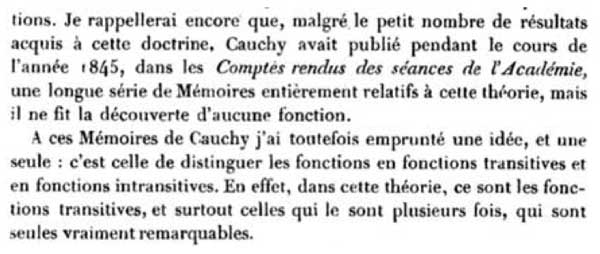Also the opening sentenses of his 1873 paper are nice, something along the lines of “if no expert was able to fill in the details of my claims made twelve years ago, I’d better do it myself”.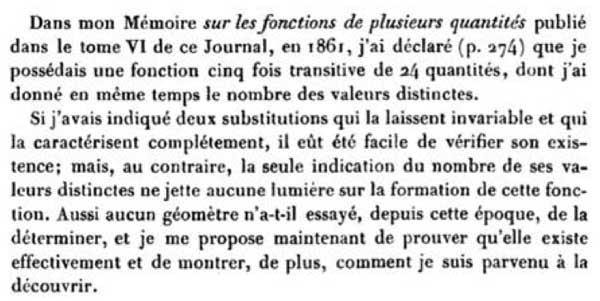However, even after this paper opinions remained divided on the issue whether or not he did really achieve his goal, and the matter was settled decisively by Ernst Witt connecting the Mathieu groups to Steiner systems (if I recall well from Mark Ronan’s book Symmetry and the monster)

As Mathieu observed, the quickest way to describe these groups would be to give generators, but as these groups are generated by two permutations on 12 respectively 24 elements, we need to have a mnemotechnic approach to be able to reconstruct them whenever needed.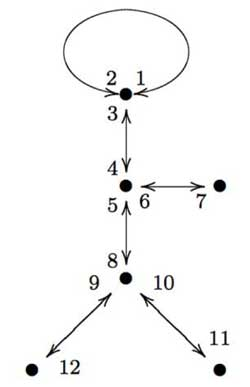Here is a nice approach, due to Gunther Malle in a Luminy talk in 1993 on “Dessins d’enfants” (more about them later). Consider the drawing of “Monsieur Mathieu” on the left. That is, draw the left-handed bandit picture on 6 edges and vertices, divide each edge into two and give numbers to both parts (the actual numbering is up to you, but for definiteness let us choose the one on the left). Then, $M_{12}$ is generated by the order two permutation describing the labeling of both parts of the edges

$s=(1,2)(3,4)(5,8)(7,6)(9,12)(11,10)$

together with the order three permutation obtained from cycling counterclockwise
around a trivalent vertex and calling out the labels one encounters. For example, the three cycle corresponding to the ‘neck vertex’ is $~(1,2,3)$ and the total permutation
is

$t=(1,2,3)(4,5,6)(8,9,10)$

A quick verification using GAP tells that these elements do indeed generate a simple group of order 95040.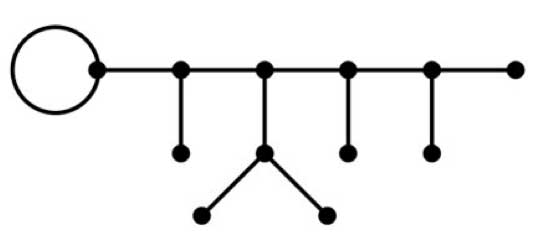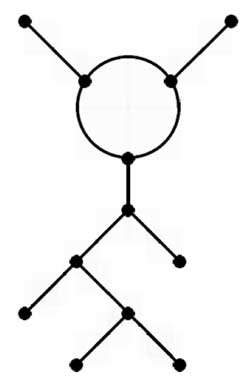Similarly, if you have to reconstruct the largest Mathieu group from scratch, apply the same method to the the picture above or to “ET Mathieu” drawing on the left. This picture I copied from Alexander Zvonkin‘s paper How to draw a group as well as the computational details below.

This is all very nice and well but what do these drawings have to do with Grothendieck’s “dessins d’enfants”? Consider the map from the projective line onto itself

$\mathbb{P}^1_{\mathbb{C}} \rightarrow \mathbb{P}^1_{\mathbb{C}}$

defined by the rational map

$f(z) = \frac{(z^3-z^2+az+b)^3(z^3+cz^2+dz+e)}{Kz}$

where N. Magot calculated that

$a=\frac{107+7 \sqrt{-11}}{486}, b=-\frac{13}{567}a+\frac{5}{1701}, c=-\frac{17}{9}, d=\frac{23}{7}a+\frac{256}{567}, e=-\frac{1573}{567}a+\frac{605}{1701}$

and finally

$K = -\frac{16192}{301327047}a+\frac{10880}{903981141}$

One verifies that this map is 12 to 1 everywhere except over the points ${ 0,1,\infty }$ (that is, there are precisely 12 points mapping under f to a given point of $\mathbb{P}^1_{\mathbb{C}} – { 0,1,\infty }$. From the expression of f(z) it is clear that over 0 there lie 6 points (3 of which with multiplicity three, the others of multiplicity one). Over $\infty$ there are two points, one with multiplicity 11 and one with multiplicity one. The difficult part is to compute the points lying over 1. The miraculous fact of the given values is that

$f(z)-1 = \frac{-B(z)^2}{Kz}$

where

$B(z)=z^6+\frac{1}{11}(10c-8)z^5+(5a+9d-7c)z^4+(2b+4ac+8e-6d)z^3+$
$(3ad+bc-5e)z^2+2aez-be)$

and hence there are 6 points lying over 1 each with mutiplicity two.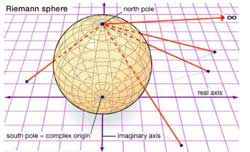Right, now consider the complex projective line $\mathbb{P}^1_{\mathbb{C}}$ as the Riemann sphere $S^2$ and mark the six points lying over 1 by a white vertex and the six points lying over 0 with a black vertex (in the source sphere). Now, lift the real interval $[0,1]$ in the target sphere $\mathbb{P}^1_{\mathbb{C}} = S^2$ to its inverse image on the source sphere. As there are exactly 12 points lying over each real
number $0 \lneq r \lneq 1$, this inverse image will consist of 12 edges which are noncrossing and each end in one black and one white vertex. The obtained graph will look like the \”Monsieur Mathieu\” drawing above with the vertices corresponding to the black vertices and the three points over 1 of multiplicity three corresponding to the
trivalent vertices, those of multiplicity one to the three end-vertices. The white vertices correspond to mid-points of the six edges, so that we do get a drawing with twelve edges, one corresponding to each number. From the explicit description of f(z) it is clear that this map is defined over $\mathbb{Q}\sqrt{-11}$ which is also the
smallest field containing all character-values of the Mathieu group $M_{12}$. Further, the Galois group of the extension $Gal(\mathbb{Q}\sqrt{-11}/\mathbb{Q}) = \mathbb{Z}/2\mathbb{Z}$ and is generated by complex conjugation. So, one might wonder what would happen if we replaced in the definition of the rational map f(z) the value of a by $a = \frac{107-\sqrt{-11}}{486}$. It turns out that this modified map has the same properties as $f(z)$ so again one can draw on the source sphere a picture consisting of twelve edges each ending in a white and black vertex.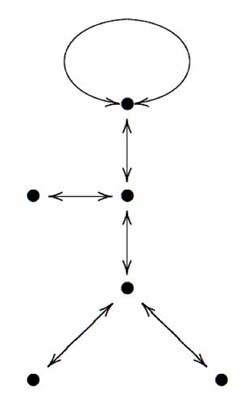If we consider the white vertices (which incidentally each lie on two edges as all points lying over 0 are of multiplicity two) as mid-points of longer edges connecting the
black vertices we obtain a drawing on the sphere which looks like \”Monsieur Mathieu\” but this time as a right handed bandit, and applying our mnemotechnic rule we obtain _another_ (non conjugated) embedding of $M_{12}$ in the full symmetric group on 12 vertices.

What is the connection with $SL_2(\mathbb{Z})$-representations? Well, the permutation generators s and t of $M_{12}$ (or $M_{24}$ for that matter) have orders two and three, whence there is a projection from the free group product $C_2 \star C_3$ (here $C_n$ is just the cyclic group of order n) onto $M_{12}$ (respectively $M_{24}$). Next
time we will say more about such free group products and show (among other things) that $PSL_2(\mathbb{Z}) \simeq C_2 \star C_3$ whence the connection with $SL_2(\mathbb{Z})$. In a following lecture we will extend the Monsieur Mathieu example to
arbitrary dessins d\’enfants which will allow us to assign to curves defined over $\overline{\mathbb{Q}}$ permutation representations of $SL_2(\mathbb{Z})$ and other _cartographic groups_ such as the congruence subgroups $\Gamma_0(2)$ and
$\Gamma(2)$.

Here are
my nominees for the 2006 paper of the year award in mathematics &
mathematical physics : in math.RA : math.RA/0606241
: Notes on A-infinity
algebras, A-infinity categories and non-commutative geometry. I
by

Maxim Kontsevich
and
Yan Soibelman
. Here is the abstract :

We develop
geometric approach to A-infinity algebras and A-infinity categories
based on the notion of formal scheme in the category of graded vector
spaces. Geometric approach clarifies several questions, e.g. the notion
of homological unit or A-infinity structure on A-infinity functors. We
discuss Hochschild complexes of A-infinity algebras from geometric point
of view. The paper contains homological versions of the notions of
properness and smoothness of projective varieties as well as the
non-commutative version of Hodge-to-de Rham degeneration conjecture. We
also discuss a generalization of Deligne’s conjecture which includes
both Hochschild chains and cochains. We conclude the paper with the
description of an action of the PROP of singular chains of the
topological PROP of 2-dimensional surfaces on the Hochschild chain
complex of an A-infinity algebra with the scalar product (this action is
more or less equivalent to the structure of 2-dimensional Topological
Field Theory associated with an “abstract” Calabi-Yau
manifold).

why ? : Because this paper
probably gives the correct geometric object associated to a
non-commutative algebra (a huge coalgebra) and consequently the right
definition of a map between noncommutative affine schemes. In a previous post (and its predecessors) I’ve
tried to explain how this links up with my own interpretation and since
another time. in hep-th : hep-th/0611082 : Children’s Drawings From
Seiberg-Witten Curves
by Sujay K. Ashok, Freddy Cachazo, Eleonora
Dell’Aquila. Here is the abstract :

We consider N=2
supersymmetric gauge theories perturbed by tree level superpotential
terms near isolated singular points in the Coulomb moduli space. We
identify the Seiberg-Witten curve at these points with polynomial
equations used to construct what Grothendieck called “dessins
d’enfants” or “children’s drawings” on the Riemann
sphere. From a mathematical point of view, the dessins are important
because the absolute Galois group Gal(\bar{Q}/Q) acts faithfully on
them. We argue that the relation between the dessins and Seiberg-Witten
theory is useful because gauge theory criteria used to distinguish
branches of N=1 vacua can lead to mathematical invariants that help to
distinguish dessins belonging to different Galois orbits. For instance,
we show that the confinement index defined in hep-th/0301006 is a Galois
invariant. We further make some conjectures on the relation between
Grothendieck’s programme of classifying dessins into Galois orbits and
the physics problem of classifying phases of N=1 gauge theories.

why ? : Because this paper gives the
best introduction I’ve seen to Grothendieck’s dessins d’enfants
(slightly overdoing it by giving a crash course on elementary Galois
theory in appendix A) and kept me thinking about dessins and their
Galois invariants ever since (again, I’ll come back to this later).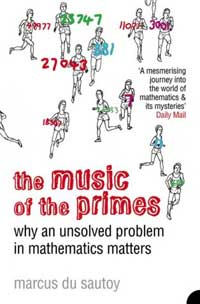Let me
admit it : i was probably wrong in this post to
garden
by Alain Connes and Matilde Marcolli. After all, it seems
that Alain&Matilde are on the verge of proving the biggest open
problem in mathematics, the Riemann
hypothesis
using noncommutative geometry. At least, this is the
impression one gets from reading through The music of the
primes, why an unsolved problem in mathematics matters
by Oxford
mathematician Prof.
Marcus du Sautoy
… At the moment I’ve only read the first
chapter (_Who wants to be a millionaire?_) and the final two
chapters (_From orderly zeros to quantum chaos_ and _The
missing piece of the jigsaw_) as I assume I’ll be familiar with most
of the material in between (and also, I’m saving these chapters for some
vacation reading). From what I’ve read, I agree most with the final
review at amazon.co.uk

Fascinating
and infuriating
, October 5, 2004
Reviewer: pja_jennings
from Southampton, Hants. United Kingdom
This is a book I found
fascinating and infuriating in turns. It is an excellent layman’s
history of number theory with particular reference to prime numbers and
the Riemann zeta function. As such it is well worth the reading.
However I found that there are certain elements, more of style than
anything else, that annoyed me. Most of the results are handed to us
without any proof whatsoever. All right, some of these proofs would be
obviously well beyond the layman, but one is described as being
understandable by the ancient Greeks (who started the whole thing) so
why not include it as a footnote or appendix?
Having established
fairly early on that the points where a mathematical function
“reaches sea level” are known as zeros, why keep reverting
to the sea level analogy? And although the underlying theme throughout
the book is the apparent inextricable link between the zeta function’s
zeros and counting primes, the Riemann hypothesis, I could find no
clear, concise statement of exactly what Riemann said.
Spanning
over 2000 years, from the ancient Greeks to the 21st century, this is a
book I would thoroughly recommend.

Books on Fermat’s last
theorem
(and there are some nice ones, such as Alf Van der Poorten’s
Notes on
Fermat’s last theorem
) can take Wiles’ solution as their focal
point. Failing a solution, du Sautoy constructs his book around an
April’s Fool email-message by Bombieri in which he claimed that a young
physicist did prove the Riemann hypothesis after hearing a talk by Alain
Connes. Here’s du Sautoy’s account (on page 3)

According
to his email, Bombieri has been beaten to his prize. ‘There are
fantastic developments to Alain Connes’s lecture at IAS last wednesday.’
Bombieri began. Several years previously, the mathematical world had
been set alight by the news that Alain Connes had turned his attention
to trying to crack the Riemann Hypothesis. Connes is one of the
revolutionaries of the subject, a benign Robespierre of mathematics to
Bombieri’s Louis XVI. He is an extraordinary charismatic figure whose
fiery style is far from the image of the staid, awkward mathematician.
He has the drive of a fanatic convinced of his world-view, and his
lectures are mesmerising. Amongst his followers he has almost cult
status. They will happily join him on the mathematical barricades to
defend their hero against any counter-offensive mounted from the ancien
regime’s entrenched positions.

Contrary to physics,
mathematics doesn’t produce many books aimed at a larger public. To a
large extend this is caused by most mathematicians’ unwillingness to
sacrifice precision and technical detail. Hence, most of us would never
be able to come up with something like du Sautoy’s description of Weil’s
work on the zeta function of curves over finite fields (page 295)

It was while exploring some of these related landscapes that
Weil discovered a method that would explain why points at sea level in
them like to be in a straight line. The landscapes where Weil was
successful did not have to do with prime numbers, but held the key to
counting how many solutions an equation such as $y^2=x^3-x$ will have if
you are working on one of Gauss’s clock calculators.

But,
it is far too easy to criticize people who do want to make the effort.
Books such as this one will bring more young people to mathematics than
any high-publicity-technical-paper. To me, the chapter on quantum chaos
was an eye-opener as I hadn’t heard too much about all of this before.
Besides, du Sautoy accompanies this book with an interesting website musicofprimes and several of
his articles for newspapers available from his homepage are
a good read (in case you wonder why the book-cover is full of joggers
with a prime number on their T-shirt, you might have a look at Beckham in his
prime number
). The music of the
primes
will definitely bring many students to noncommutative
geometry and its possible use to proving the Riemann Hypothesis.

I
expect to be writing a lot in the coming months. To start, after having
given the course once I noticed that I included a lot of new material
during the talks (mainly concerning the component coalgebra and some
extras on non-commutative differential forms and symplectic forms) so
I\’d better update the Granada notes
soon as they will also be the basis of the master course I\’ll start
next week. Besides, I have to revise the Qurves and
Quivers
-paper and to start drafting the new bachelor courses for
next academic year (a course on representation theory of finite groups,
another on Riemann surfaces and an upgrade of the geometry-101 course).

So, I\’d better try to optimize my LaTeX-workflow and learn
Here is what it is supposed to do :

pdfsync is
an acronym for synchronization between a pdf file and the TeX or so
source file used in the production process. As TeX system is not a
WYSIWYG editor, you cannot modify the output directly, instead, you must
edit a source file then run the production process. The pdfsync helps
you finding what part of the output corresponds to what line of the
source file, and conversely what line of the source file corresponds to
a location of a given page in the ouput. This feature is achieved with
the help of an auxiliary file: foo.pdfsync corresponding to a foo.pdf.

All you have to do is to put the pdfsync.sty file
in the directory _~/Library/texmf/tex/latex/pdfsync.sty_ and to
include the pdfsync-package in the preamble of the LaTeX-document. Under
my default iTex-front-end TeXShop it
works well to go from a spot in the PDF-file to the corresponding place
in the source-code, but in the other direction it only shows the
appropriate page rather than indicate the precise place with a red dot
as it does in the alternative front-end iTeXMac.

A major
drawback for me is that pdfsync doesn\’t live in harmony with my
favorite package for drawing commutative diagrams diagrams.sty. For example, the 75 pages of the current
version of the Granada notes become blown-up to 96 pages because each
commutative diagram explodes to nearly page size! So I will also have to
translate everything to xymatrix&#
8230;

The
previous part of this sequence was [quiver representations]. When $A$
is a formally smooth algebra, we have an infinite family of smooth
affine varieties $\mathbf{rep}_n~A$, the varieties of finite dimensional
representations. On $\mathbf{rep}_n~A$ there is a basechange action of
$GL_n$ and we are really interested in _isomorphism classes_ of
representations, that is, orbits under this action. Mind you, an orbit
space does not always exist due to the erxistence of non-closed orbits
so one often has to restrict to suitable representations of $A$ for
which it _is_ possible to construct an orbit-space. But first, let us
give a motivating example to illustrate the fact that many interesting
classification problems can be translated into the setting of this
non-commutative algebraic geometry. Let $X$ be a smooth projective
curve of genus $g$ (that is, a Riemann surface with $g$ holes). A
classical object of study is $M = M_X^{ss}(0,n)$ the _moduli space
of semi-stable vectorbundles on $X$ of rank $n$ and degree $0$_. This
space has an open subset (corresponding to the _stable_ vectorbundles)
which classify the isomorphism classes of unitary simple representations
$\pi_1(X) = \frac{\langle x_1,\ldots,x_g,y_1,\ldots,y_g \rangle}{([x_1,y_1] \ldots [x_g,y_g])} \rightarrow U_n(\mathbb{C})$ of the
fundamental group of $X$. Let $Y$ be an affine open subset of the
projective curve $X$, then we have the formally smooth algebra $A = \begin{bmatrix} \mathbb{C} & 0 \\ \mathbb{C}[Y] & \mathbb{C}[Y] \end{bmatrix}$ As $A$ has two
orthogonal idempotents, its representation varieties decompose into
connected components according to dimension vectors $\mathbf{rep}_m~A = \bigsqcup_{p+q=m} \mathbf{rep}_{(p,q)}~A$ all of which are smooth
varieties. As mentioned before it is not possible to construct a
variety classifying the orbits in one of these components, but there are
two methods to approximate the orbit space. The first one is the
_algebraic quotient variety_ of which the coordinate ring is the ring of
invariant functions. In this case one merely recovers for this quotient
$\mathbf{rep}_{(p,q)}~A // GL_{p+q} = S^q(Y)$ the symmetric product
of $Y$. A better approximation is the _moduli space of semi-stable
representations_ which is an algebraic quotient of the open subset of
all representations having no subrepresentation of dimension vector
$(u,v)$ such that $-uq+vp < 0$ (that is, cover this open set by $GL_{p+q}$ stable affine opens and construct for each the algebraic quotient and glue them together). Denote this moduli space by $M_{(p,q)}(A,\theta)$. It is an unpublished result of Aidan Schofield that the moduli spaces of semi-stable vectorbundles are birational equivalent to specific ones of these moduli spaces $M_X^{ss}(0,n)~\sim^{bir}~M_{(n,gn)}(A,\theta)$ Rather than studying the moduli spaces of semi-stable vectorbundles $M^{ss}_X(0,n)$ on the curve $X$ one at a time for each rank $n$, non-commutative algebraic geometry allows us (via the translation to the formally smooth algebra $A$) to obtain common features on all these moduli spaces and hence to study $\bigsqcup_n~M^{ss}_X(0,n)$ the moduli space of all semi-stable bundles on $X$ of degree zero (but of varying ranks). There exists a procedure to associate to any formally smooth algebra $A$ a quiver $Q_A$ (playing roughly the role of the tangent space to the manifold determined by $A$). If we do this for the algebra described above we find the quiver $\xymatrix{\vtx{} \ar[rr] & & \vtx{} \ar@(ur,dr)}$ and hence the representation theory of this quiver plays an important role in studying the geometric properties of the moduli spaces $M^{ss}_X(0,n)$, for instance it allows to determine the smooth loci of these varieties. Move on the the [next part. : http://www.neverendingbooks.org/index.php/quiver-representations.html